## How to calculate the correlation coefficient between two variables in Excel?

We usually use correlation coefficient (a value between -1 and 1) to display how strongly two variables are related to each other. In Excel, we also can use the CORREL function to find the correlation coefficient between two variables.

Note: A correlation coefficient of +1 indicates a perfect positive correlation, which means that as variable X increases, variable Y increases and while variable X decreases, variable Y decreases.
On the other hand, a correlation coefficient of -1 indicates a perfect negative correlation. As variable X increases, variable Z decreases and as variable X decreases, variable Z increases.

Method A Directly use CORREL function

Method B Apply Data Analysis and output the analysis

More tutorials about calculations in Excel

####Method A Directly use CORREL function

For example, there are two lists of data, and now I will calculate the correlation coefficient between these two variables.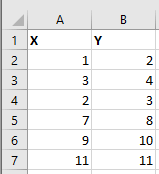Select a blank cell that you will put the calculation result, enter this formula =CORREL(A2:A7,B2:B7), and press Enter key to get the correlation coefficient. See screenshot: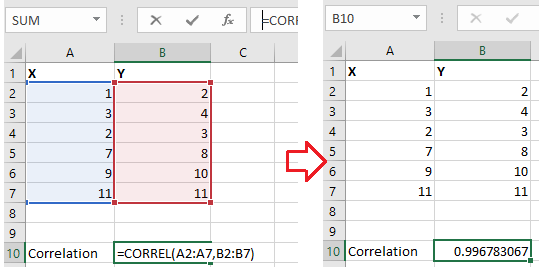In the formula, A2:A7 and B2:B7 are the two variable lists you want to compare.

you can insert a line chart to view the correlation coefficient visually. See screenshot: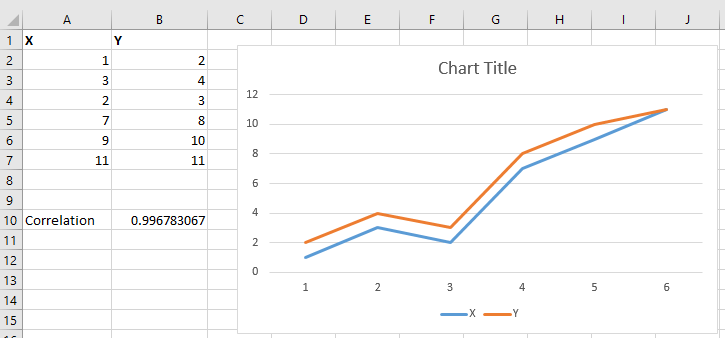#### Batch do Same Calculation on Cells

In Excel, you may want to apply the same calculation to a range of cells, generally, you will create a formula, then drag fill handle over the cells which maybe a little troublesome if the range is large. Here, the Operation Tools in Kutools for Excel, can apply one calculation to selected cells once time.
Click to have 30-day free trial with full features of Kutools for Excel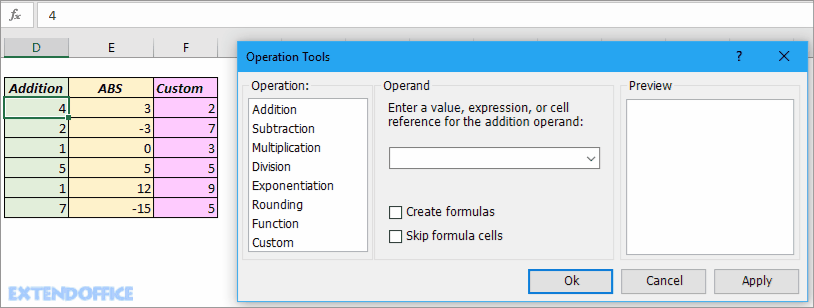####Method B Apply Data Analysis and output the analysis

With the Analysis Toolpak add-in in Excel, you can quickly generate correlation coefficients between two variables, please do as below:

1. If you have add the Data Analysis add-in to the Data group, please jump to step 3. Click File > Options, then in the Excel Options window, click Add-Ins from the left pane, and go to click Go button next to Excel Add-ins drop-down list.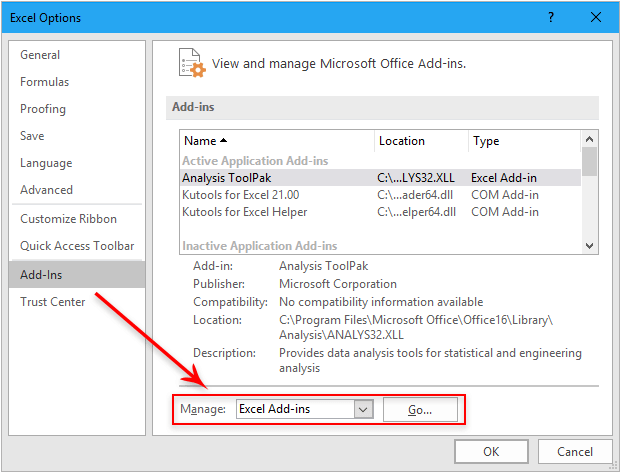2. In the Add-Ins dialog, check Analysis ToolPak, click OK to add this add-in to Data tab group.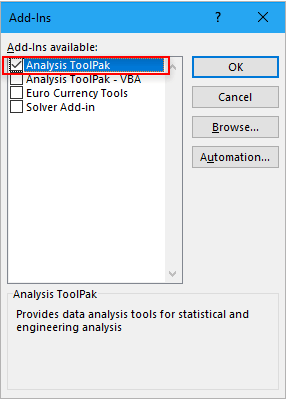3. Then click Data > Data Analysis, and in the Data Analysis dialog, select Correlation, then click OK.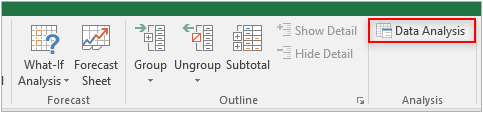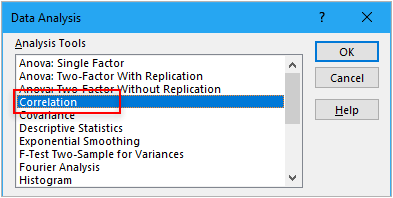4. Then the Correlation dialog, do as below operation:

1) Select the data range;

2) Check Columns or Rows option based on your data;

3) Check Labels in first row if you have labels in the data;

4) Check one option as you need in Output options secton.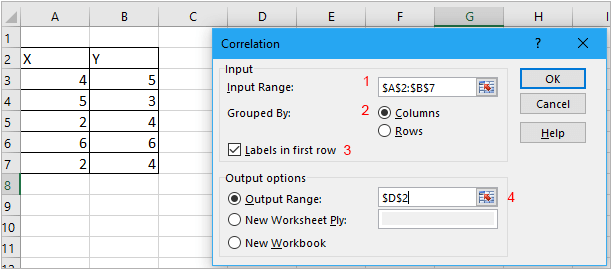5. Click OK. And the analysis result has been displayed in the range you specified.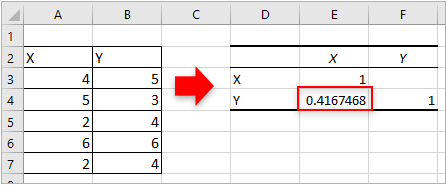#### Seconds to calculate current age based on given birthday date

If you have a list of employees' birthday, how can you quickly calculate thier current ages for each other in Excel sheet? Here the Calculate age based on birth function in Kutools for Excel can quickly solve this problem whithout remembering the complex formula.  Click for full-featured 30 days free trial!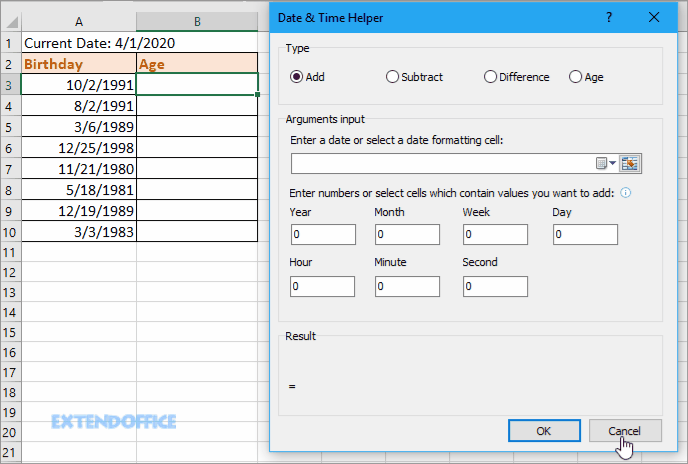Kutools for Excel: with more than 300 handy Excel add-ins, free to try with no limitation in 30 days.

#### Related Articles

• Calculate or Assign Letter Grade In Excel
To assign letter grade for each student based on their scores may be a common task for a teacher. For example, I have a grading scale defined where the score 0-59 = F, 60-69 = D, 70-79 = C, 80-89 = B, and 90-100 = A, see more.
• Calculate discount rate or price in Excel
When Christmas is coming, there must be many sale promotions in shopping malls. But if the different kinds of items have different discounts, how can you calculate the discount rates or prices of the different items?

### Best Office Productivity Tools

 Popular Features: Find, Highlight or Identify Duplicates   |  Delete Blank Rows   |  Combine Columns or Cells without Losing Data   |   Round without Formula ... Super Lookup: Multiple Criteria VLookup  |   Multiple Value VLookup  |   VLookup Across Multiple Sheets   |   Fuzzy Lookup .... Advanced Drop-down List: Quickly Create Drop Down List   |  Dependent Drop Down List   |  Multi-select Drop Down List .... Column Manager: Add a Specific Number of Columns  |  Move Columns  |  Toggle Visibility Status of Hidden Columns  |  Compare Ranges & Columns ... Featured Features: Grid Focus   |  Design View   |   Big Formula Bar   |  Workbook & Sheet Manager   |  Resource Library (Auto Text)   |  Date Picker   |  Combine Worksheets   |  Encrypt/Decrypt Cells   |  Send Emails by List   |  Super Filter   |   Special Filter (filter bold/italic/strikethrough...) ... Top 15 Toolsets:  12 Text Tools (Add Text, Remove Characters, ...)   |   50+ Chart Types (Gantt Chart, ...)   |   40+ Practical Formulas (Calculate age based on birthday, ...)   |   19 Insertion Tools (Insert QR Code, Insert Picture from Path, ...)   |   12 Conversion Tools (Numbers to Words, Currency Conversion, ...)   |   7 Merge & Split Tools (Advanced Combine Rows, Split Cells, ...)   |   ... and more

Supercharge Your Excel Skills with Kutools for Excel, and Experience Efficiency Like Never Before. Kutools for Excel Offers Over 300 Advanced Features to Boost Productivity and Save Time.  Click Here to Get The Feature You Need The Most...#### Office Tab Brings Tabbed interface to Office, and Make Your Work Much Easier

• Enable tabbed editing and reading in Word, Excel, PowerPoint, Publisher, Access, Visio and Project.
• Open and create multiple documents in new tabs of the same window, rather than in new windows.
• Increases your productivity by 50%, and reduces hundreds of mouse clicks for you every day!